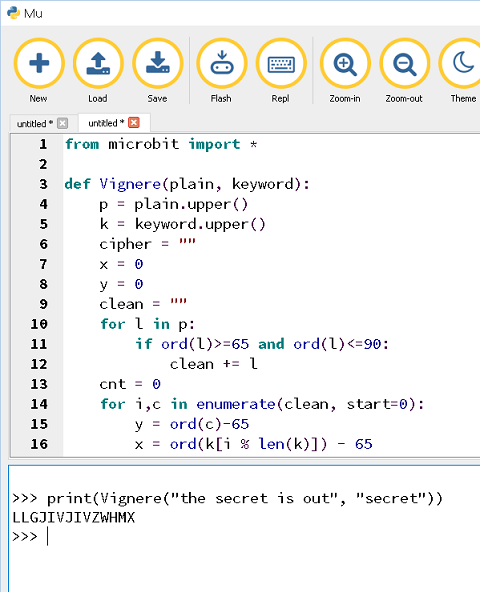# BBC micro:bit Encoding Ciphers

## Introduction

If you have written a Python program and flashed it to the micro:bit, you can use the REPL window to interact with it. This project turns the micro:bit into a cipher toolkit for encoding and decoding ciphers.

This page will start you off. There are algorithms for several ciphers in another section of this web site.

This page shows you that you can use the micro:bit as a platform for running Python programs for you to interact with via REPL.

## Caesar Shift

```from microbit import *

def Caesar(plain, shift):
cipher = ""
p = plain.upper()
for s in p:
if ord(s)==32:
cipher += " "
elif ord(s)>=65 and ord(s)<=90:
c = ord(s)
c += shift
if c<65:
c += 26
elif c>90:
c -= 26
cipher += chr(c)
return cipher
```

The program is just a function. You will need to use an REPL terminal to interact with the program. Here is an example of it in action using Mu.## Vignère

The cipher uses a keyword and more than one alphabet.

```from microbit import *

def Vignere(plain, keyword):
p = plain.upper()
k = keyword.upper()
cipher = ""
x = 0
y = 0
clean = ""
for l in p:
if ord(l)>=65 and ord(l)<=90:
clean += l
cnt = 0
for i,c in enumerate(clean, start=0):
y = ord(c)-65
x = ord(k[i % len(k)]) - 65
cipher += chr(65 + ((x+y)%26))
return cipher
```

In action,## Challenges

Have a look at some of the algorithms in the Ciphers section of the site. Just add more functions to your program and call them from the REPL window.

Keep going with this idea. You can make a whole host of functions and run them directly from the REPL window.